# Nomograms in Stata

 Disclaimer: I am not associated to StataCorp in any way. The purpose of this webpage is only to describe some useful programs and techniques for the Stata platform. > Suggested citation for nomolog and nomocox If you find these programs and/or the information included in this webpage useful for your research, please consider including the following citation: Zlotnik A, Abraira V. A general-purpose nomogram generator for predictive logistic regression models. Stata Journal. 2015. Volume 15, Number 2. > Can I get a printer-friendly version of this webpage?Yes. Click on the printer icon (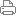) in the upper right corner of this page. Then, on the resulting webpage, clic on the same icon again to print. > What is nomolog? nomolog is a user-written program for generating nomograms for binary logistic regression predictive models. Sometimes, these are called Kattan-style nomograms. > What is nomocox? nomocox is a variation of nomolog. It generates nomograms for predictive Cox regression models. > Which versions of Stata are supported? Stata 12, Stata 13 and Stata 14. Older versions are not supported. All editions are supported (Small Stata / IC / SE / MP). Some issues may arise with Small Stata, in models with a large number of variables. Neither nomolog or nomocox are stand-alone programs. Both require Stata. > Why a Stata implementation?Stata is neither free, nor open source, however there are large discounts for students, user-written programs can extend basic capabilities and it's widely used in biomedical research. Our objective was to implement something that could work out-of-the-box, and could be used by a large number of researchers. Also, Stata is very efficient from a computational point of view (i.e. fast), a lot of attention is paid to numerical precision issues and it has a consistent syntax throughout all commands, which enables experienced users to learn new commands easily. > Who are the authors of nomolog and nomocox? Alexander Zlotnik (Technical University of Madrid & Ramón y Cajal University Hospital) and Dr. Víctor Abraira (IRICYS & Clinical Biostatistics Unit, Ramón y Cajal University Hospital). > Are these programs free to use? Yes. > How can I get help / report a bug / suggest a new feature? 1) Have a look at this webpage. 2) Have a look at the official documentation for these commands (install the programs and then execute help nomolog or help nomocox). 3) If this does not solve your question, contact me. > What are logistic and Cox regression nomograms useful for? Why would I want to use nomograms? Why should I use nomolog and nomocox? - Nomograms allow calculating output probabilities for predictive models with a visual approach. This is useful when presenting the results of your predictive models in a convenient way in a printed format. Nomograms are better than most alternative approaches, such as providing the full regression formula or a table with all regression coefficients. Another possibility is to provide an on-line calculator, but this requires some programming and usually also "hides" the underlying model, while with a nomogram, the process is fully transparent. - With nomograms, variable importance is clear at-a-glance. The longer the line corresponding to a given variable, the more important a variable is. Therefore, nomograms can also be used in descriptive or exploratory data analysis.- Nomograms can be useful for teaching purposes. Linear regressions have an intuitive interpretation. Logistic and Cox regressions are harder to understand. Most people are visual learners and providing a graphical representation can help "demystifying" these regressions. In fact, the nomograms generated with nomolog and nomocox separate the calculation mechanisms in two clear steps: (i) calculation of the "linear predictor", (ii) transformation of the "linear predictor" into a probability of event.- In our opinion, nomolog and nomocox are easy to use compared to other software packages with similar capabilities. - The resulting nomograms can be easily customized (using Stata's graph editor), as opposed to alternative implementations. Also, the resulting graphics can be stored in high quality vector format ("infinite resolution") graphics, which are often required in scientific journals. - We also believe that both nomolog and nomocox handle all possible two-variable interactions gracefully (Categorical x Continuous, Categorical x Categorical, Continuous x Continuous). > What do I need to know about Stata to use nomolog and nomocox? This tutorial is mostly self-explanatory, however you can review the following concepts: - How to use interaction variables [optional, only if you plan to use nomograms for models with interactions] > How to install the Stata Journal version of nomolog If you wish to use an older version of nomolog, which appeared in the stata Journal, you should use these commands: .net from http://www.stata-journal.com/software .net cd sj15-2 .net describe st0391 .net install st0391 > How to install the latest version of -nomolog- There is an updated version of nomolog with several modifications, which is slightly different from the Stata Journal version. 1) Open Stata 2) Make sure that you have an active Internet connection. If you are behind a proxy (f.ex. if you are connecting from your workplace or your research center), you should follow these instructions. 3) Execute .ssc install nomolog [Note: not available yet on the SSC repository. If you wish to use a pre-release version, please contact me and I'll send you the program.] > How to install the latest version of -nomocox- Follow the same steps 1) and 2) as with the nomolog and 3) Execute .ssc install nomocox [Note: not available yet on the SSC repository. Still in a beta stage. If you wish to use a pre-release version, please contact me and I'll send you the program.] > How do I add nomolog and/or nomocox to the menu?You can do this in the following way:Add nomolog to the user menu:.window menu append item "stUserGraphics" "&Nomogram post logistic regression" "db nomolog"Add nomocox to the user menu:.window menu append item "stUserGraphics" "&Nomogram post Cox regression" "db nomocox"Then, you must execute.window menu refreshIf you want to avoid writing these commands manually within each execution of Stata, add these lines to the profile.do file. > How to uninstall nomocox and nomolog Using these commands: .ado uninstall nomolog .ado uninstall nomocox > What is a nomogram? Nomograms are graphical calculators. Perhaps one of the best formal definitions we have seen is this one: Nomograms are one of the simplest, easiest and cheapest methods of mechanical calculus. (...) precision is similar to that of a logarithmic ruler (...). Nomograms can be used for research purposes (...) sometimes leading to new scientific results. Source: "Nomography and its applications" G.S.Jovanovsky, Ed. Naúka, 1977, USSR. Nomograms are widely used in several engineering disciplines. The Mizuhashi-Volpert-Smith chart, used in Electrical Engineering, is an example of a nomogram. Many non-linear functions can be represented as nomograms. Such is also the case with logistic and Cox regressions. > What is a Kattan-style nomogram? Although several nomograms have been used in biomedical research, Kattan nomograms are perhaps the most popular ones. One of the researchers who made several high-profile publications with logistic and Cox regression nomograms is Michael Kattan, PhD. You can generate Kattan-style nomograms with nomolog and nomocox. From now on, we will refer to these nomograms as logistic regression nomograms and Cox regression nomograms. You can find more details about the specific nomograms that Kattan developed here. You can see an example of a Kattan nomogram here. > How does a logistic regression nomogram look like? Example of a logistic regression nomogram:> How is a logistic regression nomogram used? Step 1) Establish scores for all variable values. Step 2) Obtain the Total score adding up all the scores obtained in the previous step. Step 3) Obtain the probability of event (Total Score -> Probability of event). Example: What is the probability of union affiliation for a worker who has a tenure of 7.5 years, works 30 hours per week, is 50 years old, is a college graduate and white? Step 1) Establish scores for all variable values. - Job tenure (years) = 7.50 => Score ≈ 3 - Usual hours worked = 30 => Score ≈ 2 - Age in current year = 50 => Score ≈ 2.5 - College graduate = yes / college grad => Score ≈ 4.2 - Race = white => Score ≈ 0Step 2) Obtain the Total score adding up all the scores obtained in the previous step. Total score = 3 + 2 + 2.5 + 4.2 + 0 = 11.7 Step 3) Obtain the probability of event (Total Score -> Probability of event). Total score =11.7 is equivalent to a probability of approximately 0.28-0.29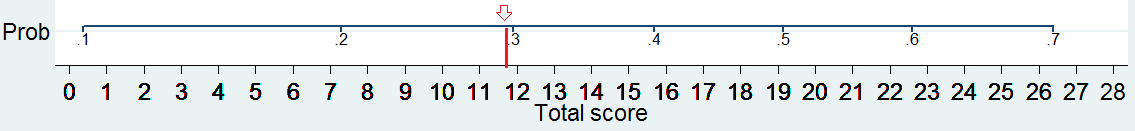> A (gentle) word of warning - A nomogram is a representation of a predictive model, not a validation tool.  If the underlying model is not adequately calibrated, the output probabilities which you may obtain with a nomogram will be generally meaningless. You must ensure that the calibration (goodness-of-fit) of the model is adequate for your purposes on at least one representative validation dataset before generating a nomogram. Ideally, calibration should be tested on several representative validation datasets. One popular method for estimating calibration is the Hosmer-Lemeshow test. You may also use the visual inspection of the Homser-Lemeshow "deciles of risk" and/or calibration graphs and/or another methods. On a side note, if in a given model calibration is not adequate but discrimination is, you may set a decision boundary for a certain probability value (ex. P = 0.5) and use the nomogram for discrimination only (decide yes if P>=0.5 and no if P<0.5).Sometimes, we are asked to implement some model validation procedure in -nomolog- / -nomocox-. We feel that this is not the purpose of these programs, as they have no reliable way of knowing if the underlying model is reasonable for your purposes. -nomolog- / -nomocox- could examine confidence intervals on the derivation dataset and complain if these were too wide, but how wide is too wide? They could also estimate calibration on the derivation dataset, but this, again, is subjective. Maybe the purpose of a particular nomogram is to produce a rough prediction (for example just three categories: likely / average / not likely) or maybe it is used for discrimination purposes only (as we stated earlier, it is possible to set a cut-off point and decide "yes/no"). This is why we believe that model validation is best left to the end-user.- Try not to generate nomograms for models with convergence problems. If you try to build a nomogram with a model where, say, some coefficients are omitted to achieve convergence, the nomogram will be generated with a warning message, however, it's best to remove the offending variable(s) and re-generate the nomogram. Although nomolog and nomocox have undergone some testing, missing coefficients may result in a representation which is not coherent with the underlying model.- Nomograms should only be used for approximate calculations. If high precision results are required, you should use an alternative approach. It must be said that this is not very common in biomedical research, mainly, because the underlying models themselves are usually not that precise. > Why are coefficients forced positive? Even if some coefficients are negative in your logistic or Cox regression, nomolog and nomocox force them positive in order to facilitate calculations (i.e. so that a nomogram can be used adding scores up only, instead of adding and subtracting scores). This is not done if interactions are used. In this case, it is up to the user to find a model wherein all coefficients are positive. > Why are variables limited to 10 labels and why is there pagination if more than 10 variables are used? This has to do with a hard-coded limitation in some graphics generation code within Stata core libraries, which cannot be modified. If too many labels are used, Stata yields an error and stops the execution. This limitation may or may not disappear in future Stata versions.  > Basic usage of nomolog 1) Open Stata 2) Load a dataset Example: .net use datasetx [Note: datasetx does not actually exist; this command is used for illustrative purposes only] 3) Execute a logistic regression Example: .logistic outcome i.varcat1 b3.varcar2 varcont b1.varcat3##varcont2 [Note: interactions are supported. b1.varcat3##varcont2 is an interaction between a categorical variable and a continuous variable] 4) Execute nomolog without any options .nomolog This will generate the nomogram with the default options. Coefficients will be forced positive to facilitate calculations. Or, alternatively, execute nomolog with execution options which allow nomogram customization. You may use the command line or just use the dialog box. .db nomolog You should see the following dialog box: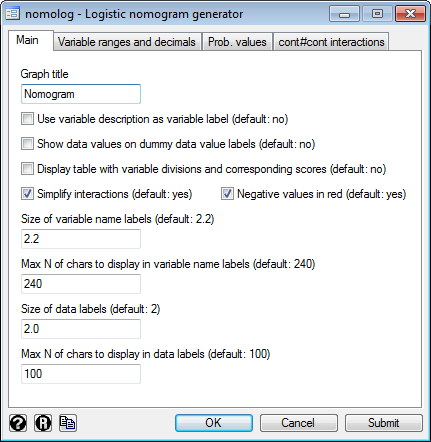Then, you can press the "OK" button to generate the nomogram. You may also change the different option to modify the resulting nomogram. > Basic usage of nomocox Steps 1) and 2) are identical to those of nomolog. 3) Set a panel variable and execute a Cox regression Example: .stset timevar .stcox outcome i.varcat1 b3.varcar2 varcont b1.varcat3##i.varcont2 4) Open the dialog box for nomocox .db nomocox You should see the following dialog box:Go to the "Survival lines" tab.Specify at least one value for S(t=value). In this example, we have used S(t=10). As you can see, up to four values can be specified.You may also specify the label for the time units ("months" in this case). Please notice that this will not change the time variable in any way, it will only change the label. It is up to the user to ensure that the labels are consistent with the actual units of the panel variable.Then, you can press the "OK" button to generate the nomogram. You may also change the different option to modify the resullting nomogram. > A simple example with nomolog .sysuse nlsw88, clear .logit union i.race i.collgrad age hours tenure [Note: logit displays regression coefficients, instead of odd ratios]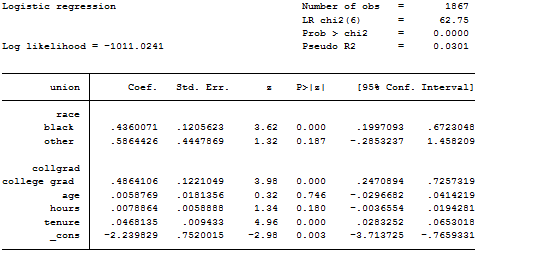.nomolog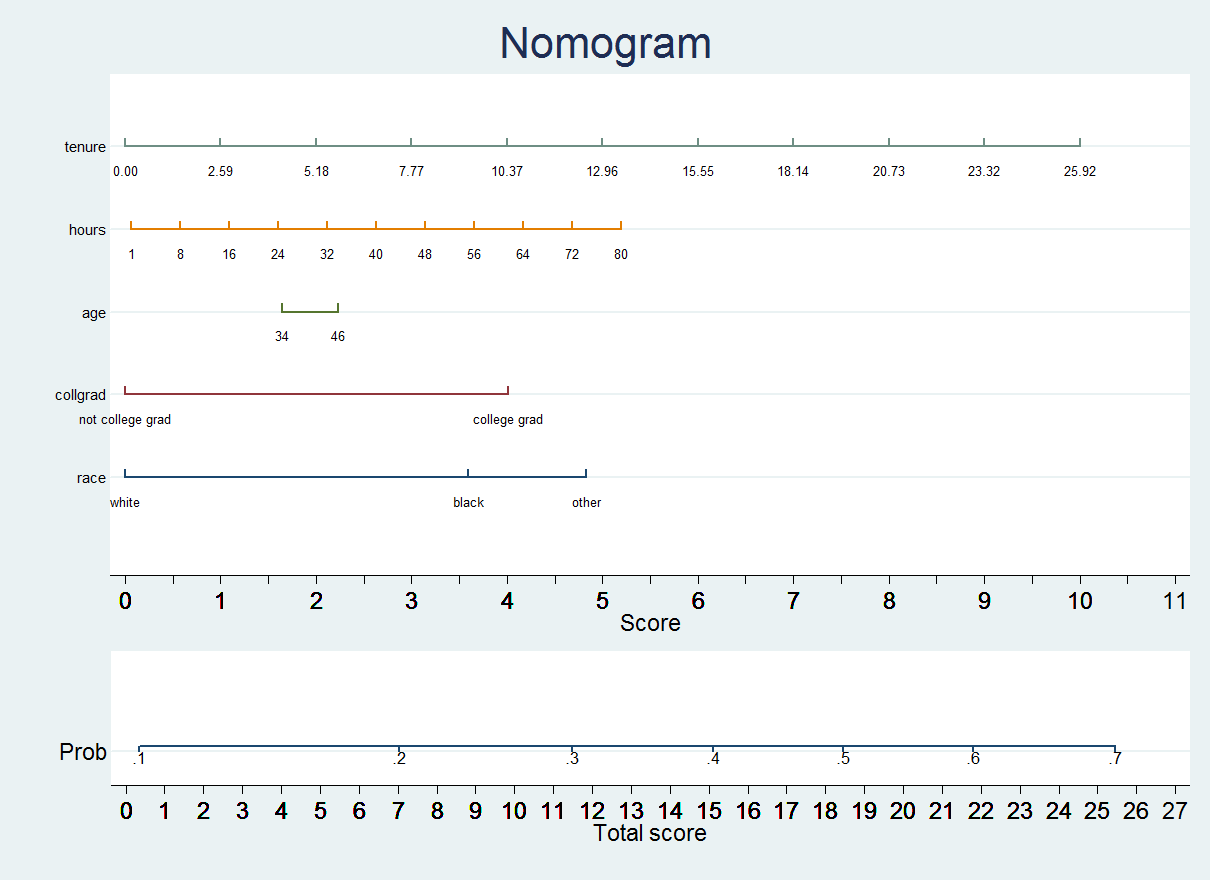> A simple example with nomocoxWe'll borrow a dataset from this webpage: http://www.ats.ucla.edu/stat/stata/seminars/stata_survival/(University of California, Los Angeles - UCLA).use http://www.ats.ucla.edu/stat/data/uis.dta, clear .stset time, failure(censor).stcox age ndrugtx i.treat i.site.db nomocox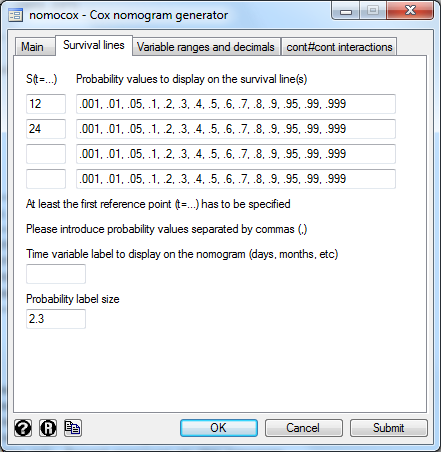This generates the command: .nomocox, s1(12) s2(24)The resulting nomogram is: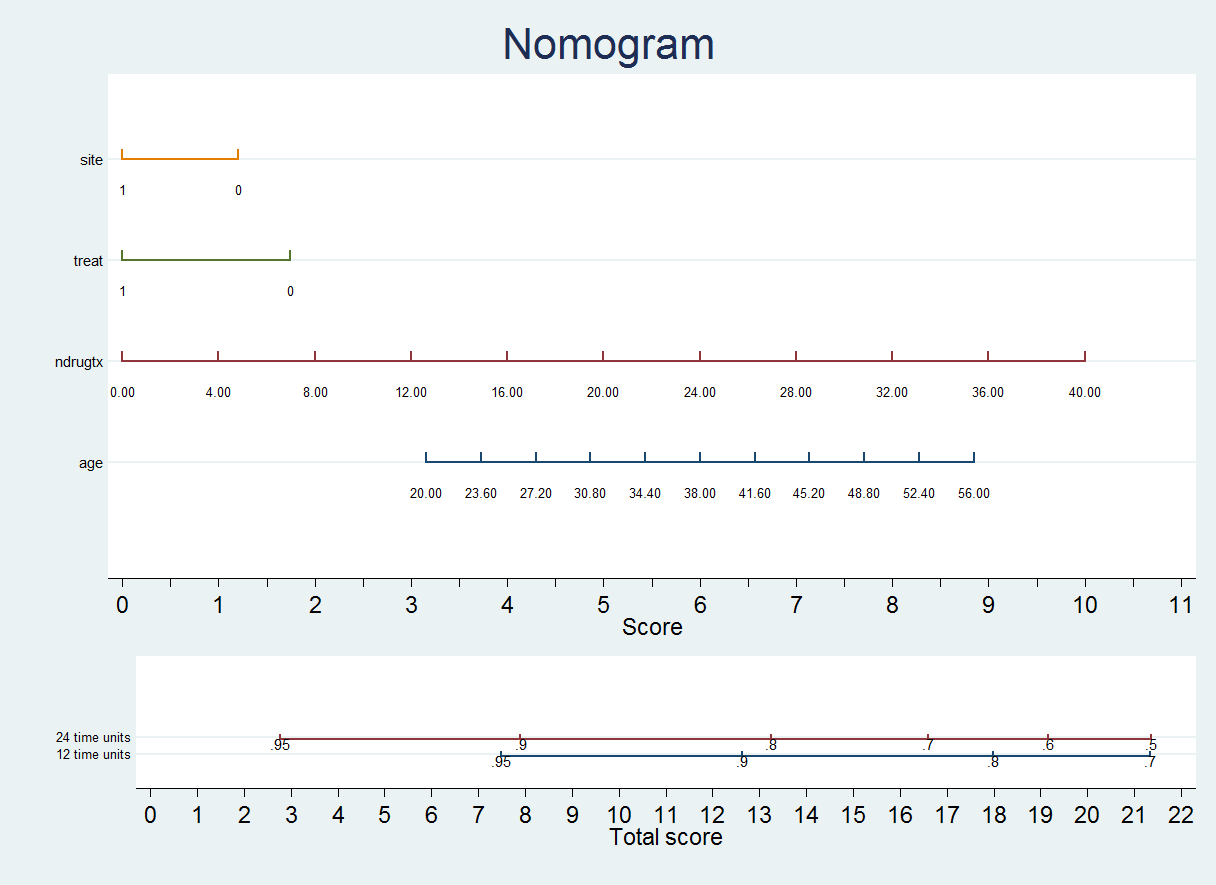The usage of a Cox regression nomogram is very similar to that of a logistic regression nomogram: Step 1) Get the scores for all variables values Step 2) Add the scores = Total score Step 3) Calculated the probability of survival for a given number of time units given the Total scoreExample: in this nomogram a Total score = 8 implies - an approximate probability of survival for 12 time units of .946-.948- an approximate probability of survival for 24 time units of .9 > Interactions in nomograms Both nomolog and nomocox support interactions. An interaction is a product of variables, i.e. interaction_variable = var_A * var_BThe syntax to include an interaction term in Stata is interaction_variable = var_A#var_BAn interaction variable can be included directly in a logistic or a Cox regression command:.logit outcome var1#var2.stcox outcome var1#var2There are three possibilities for interactions in nomolog and nomocox: 1) Categorical x CategoricalExample: .logit outcome i.var1#i.var2.nomolog 2) Categorical x ContinuousExample:.logit outcome i.var1#c.var2.nomolog 3) Continuous x ContinuousExample:.logit outcome c.var1#c.var2.nomolog, k1(var1,10,20,30) This last case is slightly more complicated since cutpoints need to be specified on the nomogram in order to make it linear. In this particular case we are specifying cutpoints 10, 20 and 30 for var1. If we did a product of continuous variables, the resulting score calculation would not be linearMost often, full interactions (include not just i.var1#i.var2, but also i.var1 and i.var2) are included in the model, due to the "hierarchy principle".Full interactions can be generated in Stata with the ## operator (i.var1##i.var2 is equivalent to including variables i.var1#i.var2, i.var1 and i.var2)If full interactions are used, nomolog and nomocox try to simplify the nomogram.Notice that if interactions are used, it is no longer possible to force variables positive. In these cases negative coefficients are displayed in red color. > A simple example with nomocoxAgain, we'll borrow a dataset from this webpage: http://www.ats.ucla.edu/stat/stata/seminars/stata_survival/(University of California, Los Angeles - UCLA) .use http://www.ats.ucla.edu/stat/data/uis.dta, clear.stset time, failure(censor) .stcox age ndrugtx i.treat##i.site (Notice that this is equivalent to .stcox age ndrugtx i.treat#i.site i.treat i.site).db nomocoxThis generates the command: .nomocox, s1(12) s2(24) The resulting nomogram is: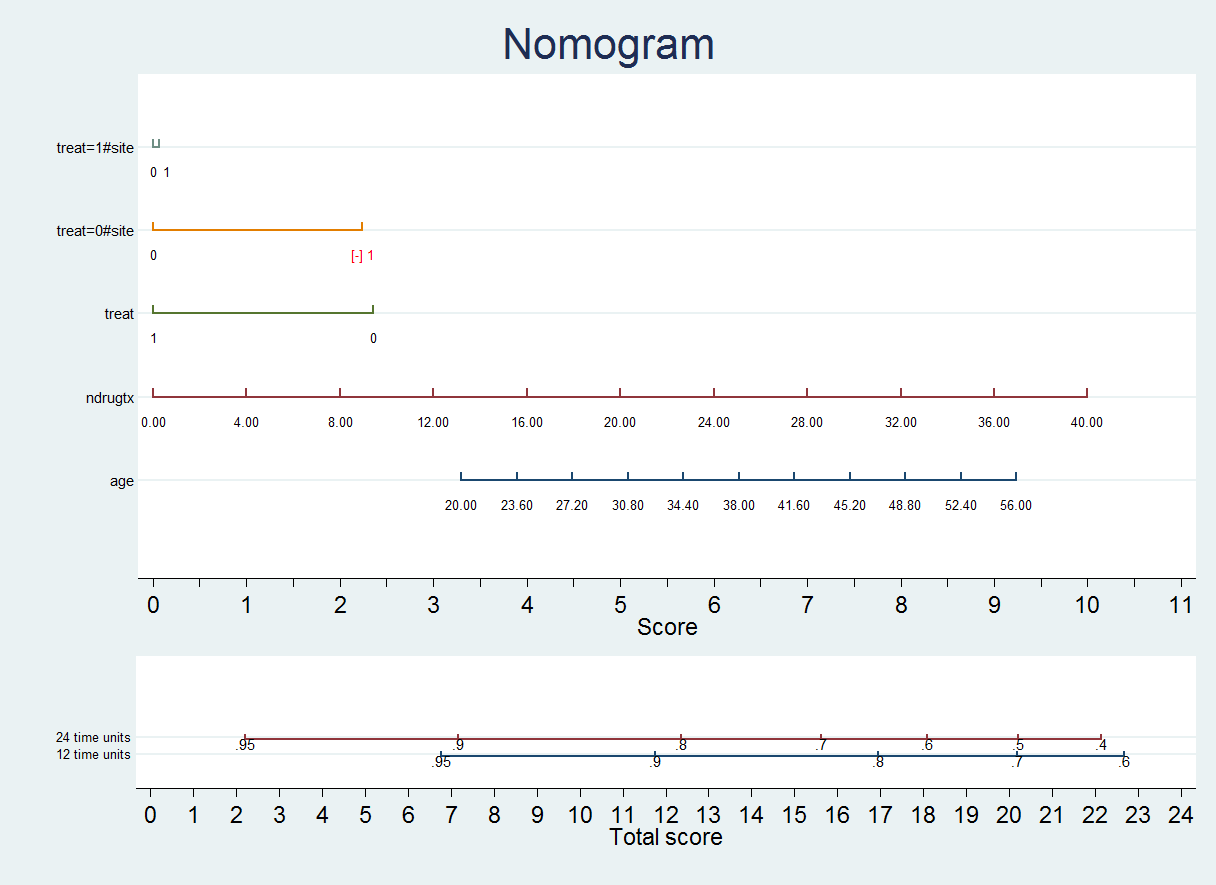We see that the variable treat has two values: 0 and 1Let's see how to calculate the scores for treat and its interaction with site. If treat = 1, we choose the first line and obtain a score depending on the value of site: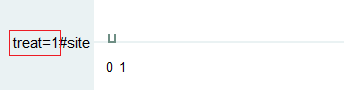This line gives us the scores for: treat=1 and site=0treat=1 and site=1If treat = 0, we choose the second line and obtain a score depending on the value of site:This line gives us the scores for: treat=0 and site=0treat=0 and site=1Notice that, in this particular case, there is a negative score if treat = 0 and site = 1 (marked with [-] and in red color)We would then calculate the score corresponding to treat: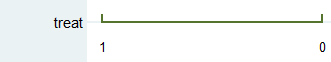By default both nomolog and nomocox simplify interactions. For example, in this case, we have removed the line corresponding to the effect of site combining it with the lines corresponding to the interaction of treat and site. However, in some cases, we may want to display all variables independently on the nomogram. .db nomocoxUncheck the "Simplify interactions" checkbox: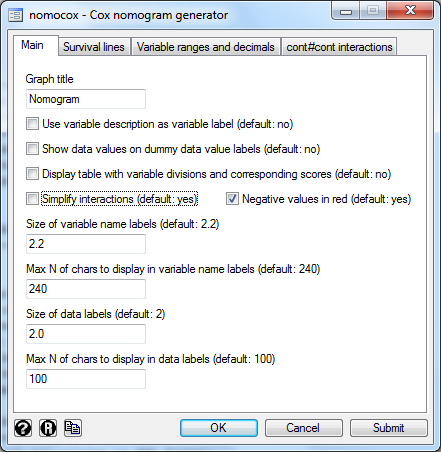The resulting nomogram is the following: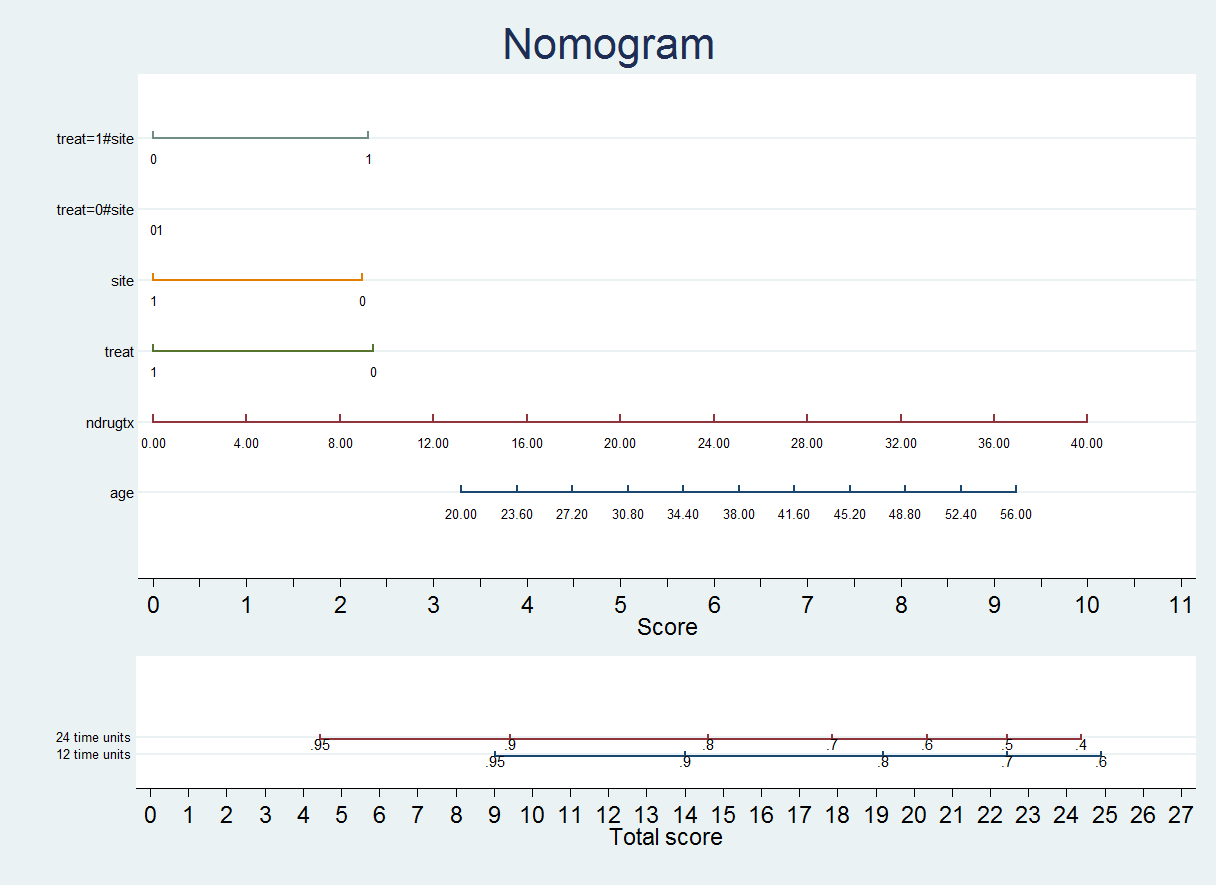Notice that in this case the score for treat=0#site is almost zero.Both nomograms (the previous one and this one) are equivalent. However, on the second one more variable has to be taken into account to make to obtain the outcome probability. > How to change variable label sizes 1) Open the dialog box. .db nomolog 2) Change the value in the corresponding box (for example 2.0):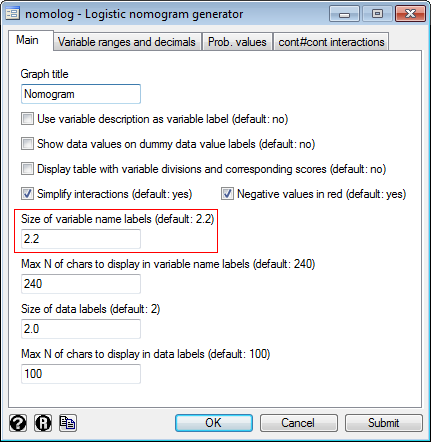3) Press the "OK" button. 4) This will result in the following command being executed: .nomolog, varlblsize(2.0) [Note: 2.0 can be replaced with any decimal value] [Note: you may also use the command directly] > How to display variable labels instead of variable names As you most likely know, Stata allows you to associate variable labels to variables. Some commands can make use of either. Example of label definition command: .label variable tenure "job tenure (years)" 1) Open the dialog box. .db nomolog 2) Mark the check box: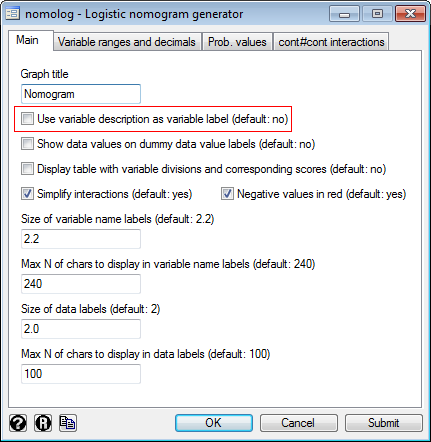3) Press the "Submit" button. 4) This will result in the following command being executed: .nomolog, varlabdescr [Note: you may also use the command directly] > How to change the size of data labels (i.e. the numbers that appear above numeric variables and the names of the categories in categorical variables) 1) Open the dialog box. .db nomolog 2) Change the value in the corresponding box (for example 1.8):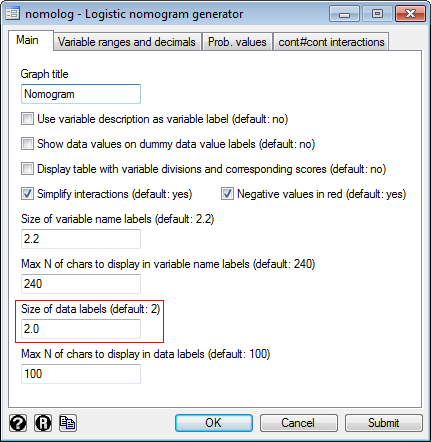3) Press the "OK" button. 4) This will result in the following command being executed: .nomolog, datalblsize(1.8) [Note: you may also use the command directly] > How to change variable ranges Sometimes, you may be interested in changing variable ranges. For example, the age values in your dataset range from 34 to 46 years. This is not very convenient for calculations and, besides, you may want to use a larger age range, for example 20 to 60 years. Warning: nomolog and nomocox allow you to do this, but be aware that if you use a more extensive variable range than the actual variable range, you will be using values which do not exist in your dataset (i.e. you would be using out-of-sample values). In the example above, is it legitimate to extend the variable range from 20 to 60 years if your dataset actually ranges from 34 to 46 years? It depends. - If this generalization is reasonable for your dataset and the model you have chosen, you may do this. - But, maybe, if you did include actual data for persons from 20 to 60 years, the coefficients of the model would be different. If you feel this may be the case, you should avoid extending a variable range artificially.[Note: if you change a variable range, a notice will be displayed on the right-bottom corner of the nomogram. You may remove it with the Stata's Graph Editor] 1) Open the dialog box. .db nomolog 2) Go to the "Variable ranges and decimals" tab. Introduce the variable(s), the new minimum(s), maximum(s) and division size(s):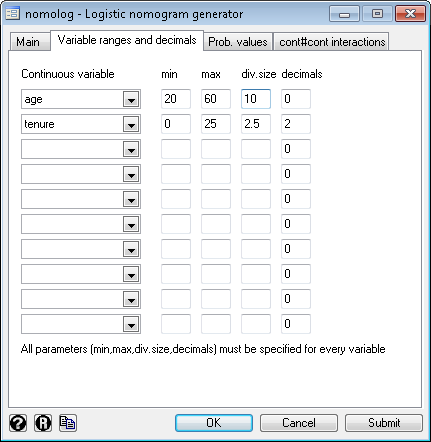3) Press the "OK" button. 4) This will result in the following command being executed: .nomolog, vli1(age,20,60,10,0) vli2(tenure,0,25,5,2) [Note: you may also use the command directly] > How to change the probability axis range and/or probability label sizes 1) Open the dialog box. .db nomolog 2) Go to the "Prob. values" tab and insert the probability values you want to display: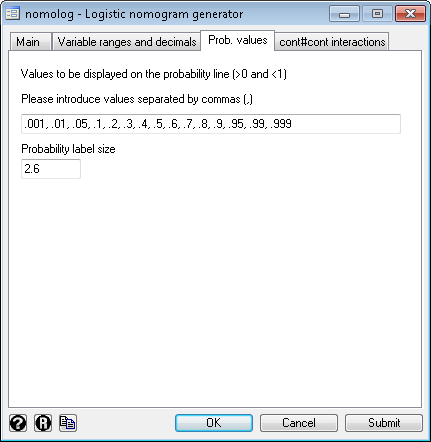Notice that here you may also change the probability label size too. 3) Press the "OK" button. 4) This will result in the following command being executed: .nomolog, prvalues(.001, .01, .05, .1, .2, .3, .4, .5, .6, .7, .8, .9, .95, .99) [Note: you may also use the command directly] > Another example of the same nomogram using several execution options .sysuse nlsw88, clear .quietly logit union i.race i.collgrad age hours tenure [Note: "quietly" hides the output] .nomolog, vli1(hours,20,80,10,0) vli2(tenure,0,25,2.5,2) vli3(age,20,60,10,0) title("Probability of union affiliation") The resulting nomogram will look like this: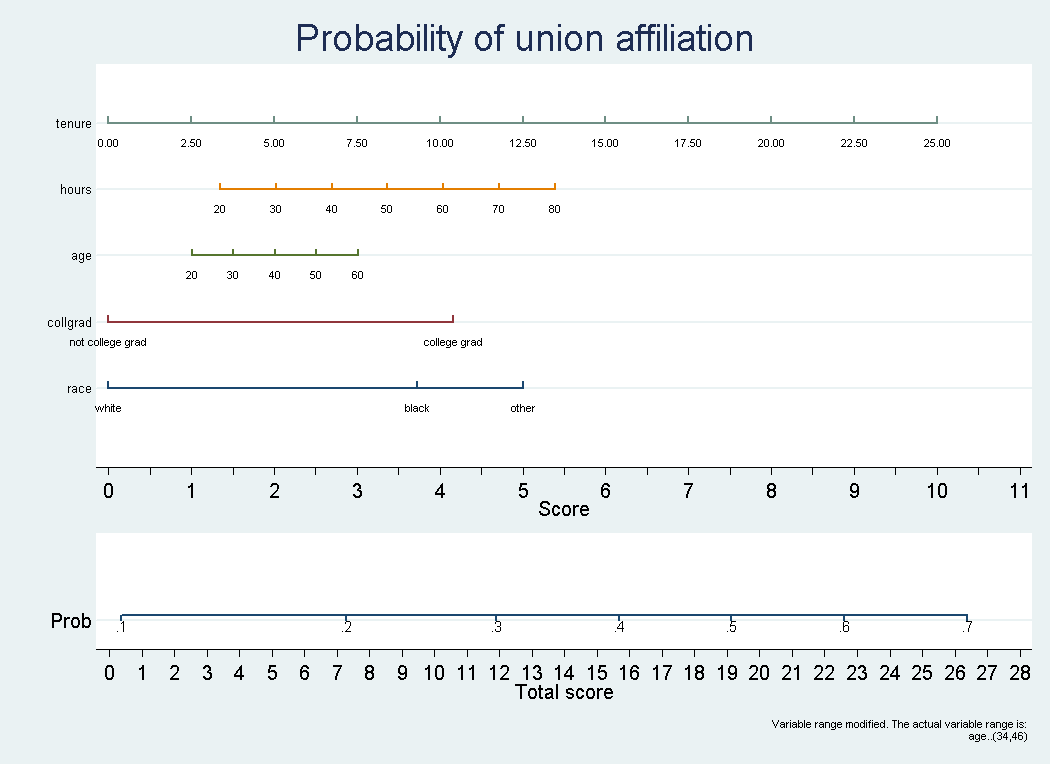Notice that, in this case, a warning has been added to the graph indicating that the range (min, max values to display) of variable age has been changed, i.e. the nomogram is actually showing an out-of-sample prediction (age values that were not in the derivation dataset). You may remove this warning using Stata's Graph Editor.Also, notice that no such warning is displayed for variables tenure and hours. This is because their respective ranges were not modified, only the number of divisions and / or the number of decimals to display. > Is it possible to generate nomograms with interactions (products) between variables? Yes! In fact, we believe that nomolog and nomocox are the only programs that easily handle nomograms with all possible two-variable interaction scenarios gracefully. There are three possibilities for two-variable interactions: - Categorical x Continuous interactions Example: .quietly logit union i.race i.collgrad##age hours tenure - Categorical x Categorical interactions Example: .quietly logit union i.race##i.collgrad age hours tenure - Continuous x Continuous interactionsExample: .quietly logit union i.race i.collgrad age##hours tenure [Note: when defining continuous x continuous interactions, reference values must be defined for one of the variables]The nomogram is generated in the same way as with a model without interactions. However, if interactions are used, the variables are not necessarily forced positive. There is an option (true by default) to simplify interactions (on the first tab of the dialog box). Unless you want to do some debugging, it's best not to change this option from its default value.[Note: higher order interactions are not supported. For example, a nomogram for a model with age##hours##i.race cannot be generated] > Is it possible to export the values of the divisions for all variables and the corresponding scores? Yes. Mark the option "Display table with variable divisions and corresponding scores" on the dialog box or use the option "divtable" if you are using the command line.This may be useful for checking the resulting nomogram and/or generating an alternative nomogram with a different Stata command or software package. > How to perform other modifications on the resulting graph(s) nomolog and nomocox generate standard Stata graphs, which can be edited with the Stata's Graph Editor. By the way, the resulting graphs can be exported to a variety of vector formats ("infinite resolution" formats), which make them adequate for the requirements of all scientific publications. > Acknowledgments We would like to thank all Stata Corp technical support personnel we had the opportunity to work with, especially Statistician Joy Wang, and all the members of the Clinical Biostatistics Unit of the Ramon y Cajal University Hospital who have participated in the testing of this program. > References - Zlotnik A, Abraira V. A general-purpose nomogram generator for predictive logistic regression models. Stata Journal. 2015. Volume 15, Number 2. - Zlotnik A, Abraira V. Development of the nomolog program and its evolution. Towards the implementation of a nomogram generator for the Cox regression. 2014 Spanish Stata Users Group Meeting. Barcelona, Spain. - Zlotnik A, Abraira V. Stata logistic regression nomogram generator. 2013 Spanish Stata Users Group Meeting. Madrid, Spain. - Fu AZ, Cantor SB, Kattan MW: Use of nomograms for personalized decision-analytic recommendations. Medical Decision Making 2010, 30(2):267-274. - Kattan MW, Wheeler TM, Scardino PT: Postoperative nomogram for disease recurrence after radical prostatectomy for prostate cancer. Journal of Clinical Oncology 1999, 17(5):1499-1499. - Kattan MW, Eastham JA, Stapleton AM, Wheeler TM, Scardino PT: A preoperative nomogram for disease recurrence following radical prostatectomy for prostate cancer. Journal of the National Cancer Institute 1998, 90(10):766-771.